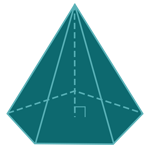Report a Problem
Suggestions

#Calculate The Surface Area of A Pentagonal Pyramid

Last updated: Saturday, April 29, 2023
Select a type of pyramid below
Rectangular Base
Square Base
Equilateral Triangular Base
Tetrahedron
Pentagonal Base
Hexagonal Base

A pentagonal pyramid is a 3-dimensional geometric shape with a pentagonal base and five triangular faces that meet at a common point at the top. It is a type of pyramid that is commonly used in mathematics, physics, and architecture. The surface area of a pentagonal pyramid can be calculated by finding the area of the base and adding it to the area of the five triangular faces.

Pentagonal pyramids are not commonly found in everyday objects, but they do have applications in architecture and design. The famous Louvre Pyramid in Paris is an example of a pentagonal pyramid, designed by architect I.M. Pei. This pyramid serves as the main entrance to the Louvre Museum, and its shape has become an iconic symbol of the museum. Pentagonal pyramids are also used in construction, such as in the design of buildings with unique architectural features or in the creation of sculptural forms. Additionally, pentagonal pyramids are used in 3D modeling and computer graphics to create complex shapes and objects.

The formula for determining the surface area of a pentagonal pyramid is defined as:
$$SA$$ $$=$$ $$\dfrac{5}{4}$$ $$\cdot$$ $$\tan(54^\circ)$$ $$\cdot$$ $$a^2$$ $$+$$ $$5$$ $$\cdot$$ $$\dfrac{a}{2}$$ $$\cdot$$ $$\sqrt{h^2 + \Big(\dfrac{a \cdot \tan(54^\circ)}{2}\Big)^2}$$
$$SA$$: the surface area of the pyramid
$$a$$: the length of any side of the base
$$h$$: the height of the pyramid
The SI unit of surface area is: $$square \text{ } meter\text{ }(m^2)$$

## Find $$SA$$

Use this calculator to determine the surface area of a pentagonal pyramid when the length of any side of the base and the height of the pyramid are given.
Hold & Drag
CLOSE
the length of any side of the base
$$a$$
$$meter$$
the height of the pyramid
$$h$$
$$meter$$
Bookmark this page or risk going on a digital treasure hunt again